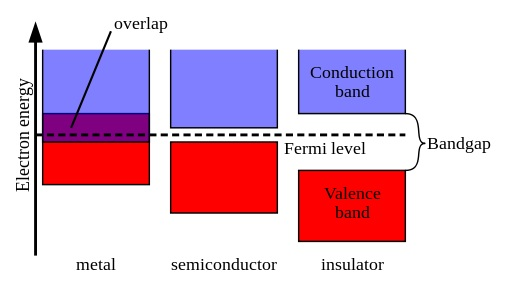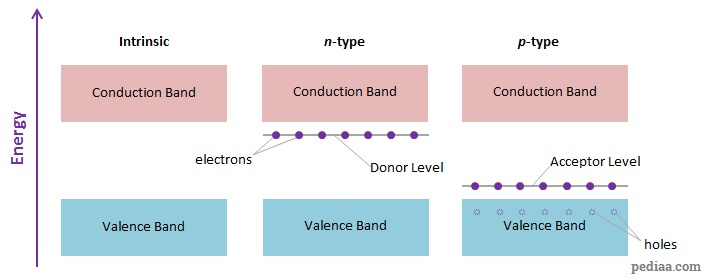# Difference Between p-type and n-type Semiconductor

## Main Difference – p-type vs. n-type Semiconductor

p-type and n-type semiconductors are absolutely crucial to the construction of modern electronics. They are very useful because their conduction abilities can be easily controlled. Diodes and transistors, which are central to all sorts of modern electronics, require p-type and n-type semiconductors for their construction. The main difference between p-type and n-type semiconductor is that p-type semiconductors are made by adding impurities of Group-III elements to intrinsic semiconductors whereas, in n-type semiconductors, the impurities are Group-IV elements.

## What is a Semiconductor

semiconductor is a material that has a conductivity between that of a conductor and an insulator. In the band theory of solids, energy levels are represented in terms of bands. Under this theory, for a material to conduct, electrons from the valence band should be able to move up to the conduction band (note that “moving up” here does not mean an electron physically moving up, but rather an electron gaining an amount of energy that is associated with the energies of the conduction band). According to the theory, metals (which are conductors) have a band structure where the valence band overlaps with the conduction band. As a result, metals can readily conduct electricity. In insulators, the band gap between the valence band and the conduction band is quite large so that it is extremely difficult for electrons to get into the conduction band. In contrast, semiconductors have a small gap between the valence and conduction bands. By increasing the temperature, for example, it is possible to give electrons enough energy that allows them to move from the valence band up to the conduction band. Then, the electrons can move in the conduction band and the semiconductor can conduct electricity.How metals (conductors), semiconductors and insulators are viewed under the band theory of solids.

Intrinsic semiconductors are elements with four valence electrons per atom, i.e. elements that occur in “Group-IV” of the periodic table such as silicon (Si) and germanium (Ge). Since each atom has four valence electrons, each of these valence electrons can form a covalent bond with one of the valence electrons in a neighbouring atom. In this way, all of the valence electrons would be involved in a covalent bond. Strictly speaking, this is not the case: depending on the temperature, a number of electrons are able to “break” their covalent bonds and take part in conduction. However, it is possible to greatly increase the conducting ability of a semiconductor by adding small quantities of an impurity to the semiconductor, in a process called doping. The impurity that is added to the intrinsic semiconductor is called the dopant. A doped semiconductor is referred to as an extrinsic semiconductor.

## What is an n-type Semiconductor

An n-type semiconductor is made by adding a small amount of a Group-V element such as phosphorous (P) or arsenic (As) to the intrinsic semiconductor. Group-V elements have five valence electrons per atom. Therefore, when theses atoms make bonds with the Group-IV atoms, due to the atomic structure of the material only four out of the five valence electrons can be involved in covalent bonds. This means that per each dopant atom there is an extra “free” electron that can then go into the conduction band and start conducting electricity. Therefore, the dopant atoms in n-type semiconductors are called donors because they “donate” electrons to the conduction band. In terms of the band theory, we can imagine the free electrons from donors having an energy level close to the energies of the conduction band. Since the energy gap is small, the electrons can easily jump into the conduction band and start conducting a current.

## What is a p-type Semiconductor

p-type semiconductor is made by doping an intrinsic semiconductor with Group-III elements such as boron (B) or aluminium (Al). In these elements, there are only three valence electrons per atom. When these atoms are added to an intrinsic semiconductor, each of the three electrons can form covalent bonds with valence electrons from three of the surrounding atoms of the intrinsic semiconductor. However, due to the crystalline structure, the dopant atom can make another covalent bond if it had one more electron. In other words, there is now a “vacancy” for an electron, and often such a “vacancy” is called a hole. The dopant atom can now take an electron out of one of the surrounding atoms and use that to form a bond. In p-type semiconductors, the dopant atoms are called acceptors since they take electrons for themselves.

Now, the atom that had an electron stolen from it is left with a hole as well. This atom can now steal an electron from one of its neighbours, which, in turn, can steal an electron from one of its neighbours… and so on. In this way, we can actually imagine that a “positively-charged hole” can travel through the valence band of a material, in much the same way that an electron can travel through the conduction band. The “movement of holes” in the conduction band can be viewed as a current. Note that the motion of holes in the valence band is in the opposite direction to the motion of electrons in the conduction band for a given potential difference. In p-type semiconductors, the holes are said to be the majority carriers while the electrons in the conduction band are the minority carriers.

In terms of band theory, the energy of the accepted electrons (“the acceptor level”) lie a little higher above the energy of the valence band. Electrons from the valence band can easily reach this level, leaving holes behind in the valence band. The diagram below illustrates the energy bands in intrinsic, n-type and p-type semiconductors.Energy bands in intrinsic, n-type and p-type semiconductors.

## Difference Between p-type and n-type Semiconductor

### Dopants

In p-type semiconductor, the dopants are Group-III elements.

In n-type semiconductor, the dopants are Group-IV elements.

### Dopant Behaviour:

In p-type semiconductor, the dopant atoms are acceptors: they take electrons and create holes in the valence band.

In n-type semiconductor, the dopant atoms act as donors: they donate electrons which can easily reach the conduction band.

### Majority Carriers

In p-type semiconductor, the majority carriers are holes which move in the valence band.

In n-type semiconductor, the majority carriers are electrons that move in the conduction band.

### Majority Carriers Movement

In p-type semiconductor, the majority carriers move in the direction of conventional current (from higher to lower potential).

In n-type semiconductor, the majority carriers move against the direction of conventional current.

Image Courtesy:

“Comparison of the electronic band structures of metals, semiconductors and insulators.” by Pieter Kuiper (self-made) [Public Domain], via Wikimedia Commons